# Newtons Laws of Motion Newtons First Law of

• Slides: 16Newton’s Laws of MotionNewton’s First Law of Motion n An object at rest tends to stay at rest, and an object in motion tends to stay in motion, unless acted upon by an outside, unbalanced force.Newton’s First Law of Motion n n Objects – whether they are staying still or moving – tend to keep on doing what they’re doing until something interferes. When we put something down, it tends to stay in that spot until someone or something moves it.Newton’s First Law of Motion n How does a moving object stay in motion? ? ? q q Can you picture an object moving forever since objects always seem to slow down at some point? ? ? Why do objects slow down? ? ?Newton’s First Law of Motion n n It is also called the “law of inertia. ” Inertia is another word to describe an object’s tendency to stay in motion or at rest unless an outside force interferes.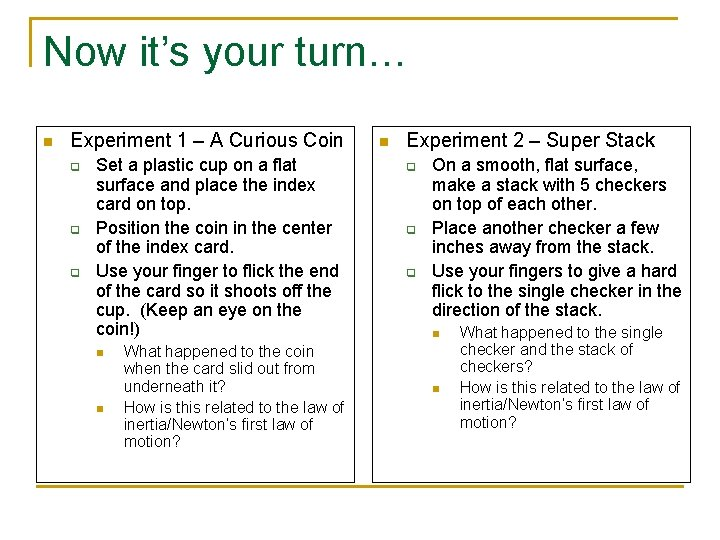Now it’s your turn… n Experiment 1 – A Curious Coin q q q Set a plastic cup on a flat surface and place the index card on top. Position the coin in the center of the index card. Use your finger to flick the end of the card so it shoots off the cup. (Keep an eye on the coin!) n n What happened to the coin when the card slid out from underneath it? How is this related to the law of inertia/Newton’s first law of motion? n Experiment 2 – Super Stack q q q On a smooth, flat surface, make a stack with 5 checkers on top of each other. Place another checker a few inches away from the stack. Use your fingers to give a hard flick to the single checker in the direction of the stack. n n What happened to the single checker and the stack of checkers? How is this related to the law of inertia/Newton’s first law of motion?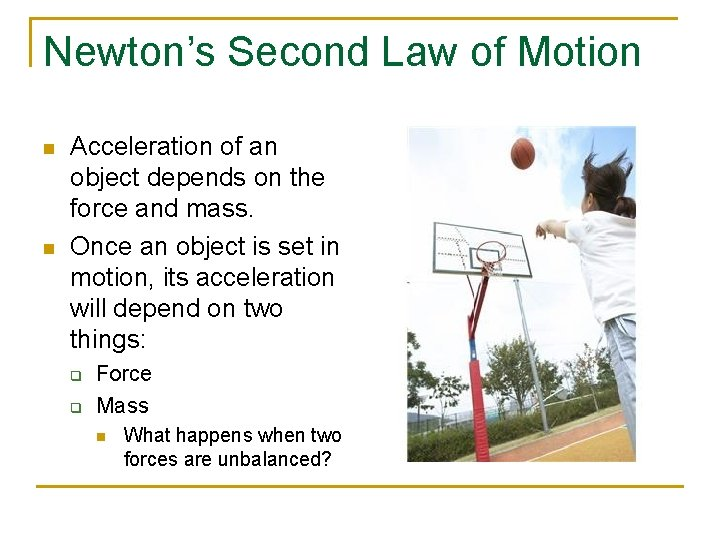Newton’s Second Law of Motion n n Acceleration of an object depends on the force and mass. Once an object is set in motion, its acceleration will depend on two things: q q Force Mass n What happens when two forces are unbalanced?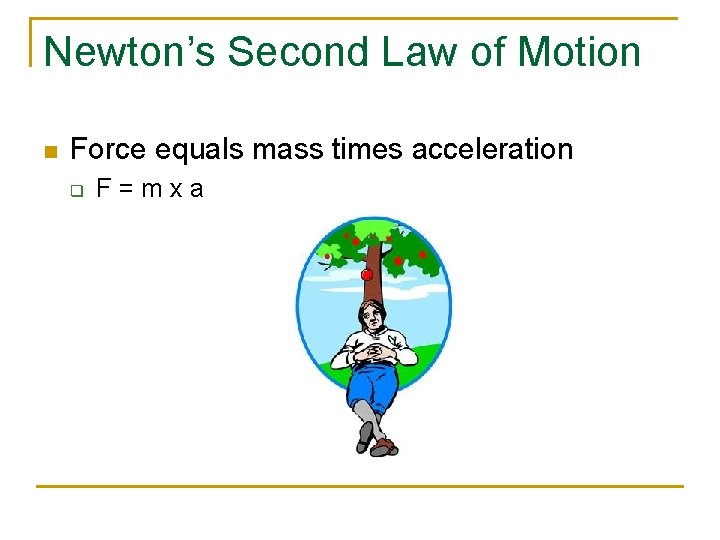Newton’s Second Law of Motion n Force equals mass times acceleration q F=mxaNewton’s Second Law of Motion n The greater the force exerted on an object, the more it will accelerate. q Ex. The harder you kick a ball, the farther and faster it will travel.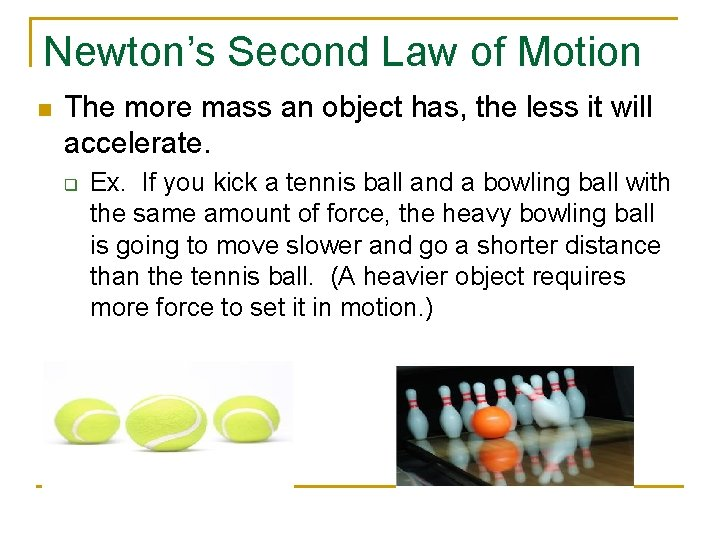Newton’s Second Law of Motion n The more mass an object has, the less it will accelerate. q Ex. If you kick a tennis ball and a bowling ball with the same amount of force, the heavy bowling ball is going to move slower and go a shorter distance than the tennis ball. (A heavier object requires more force to set it in motion. )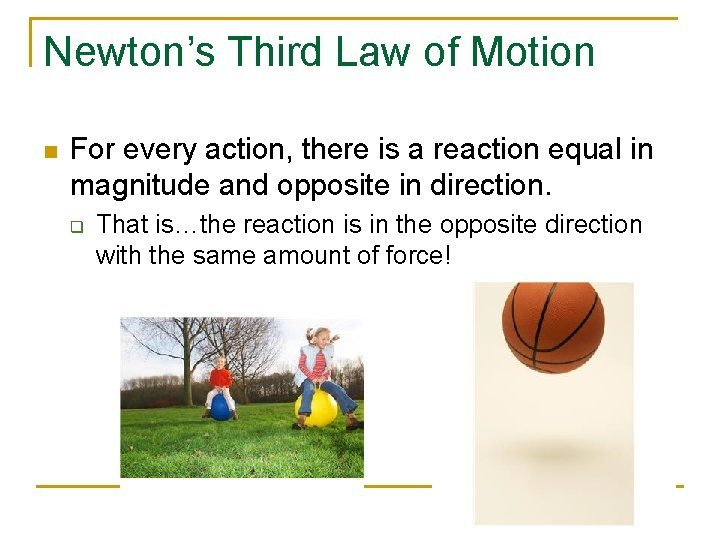Newton’s Third Law of Motion n For every action, there is a reaction equal in magnitude and opposite in direction. q That is…the reaction is in the opposite direction with the same amount of force!Newton’s Third Law of Motion n Forces always occur in pairs. Every time a force, or action, occurs, it causes a reaction. The reaction can be described by its strength and direction.Newton’s Third Law of Motion n The magnitude, or strength, of the action is equal to the magnitude of the reaction. q q For example – If you toss a pebble into the water, its going to cause a small ripple or a splash. If you hurl a large bolder at the water, the splash is going to be bigger.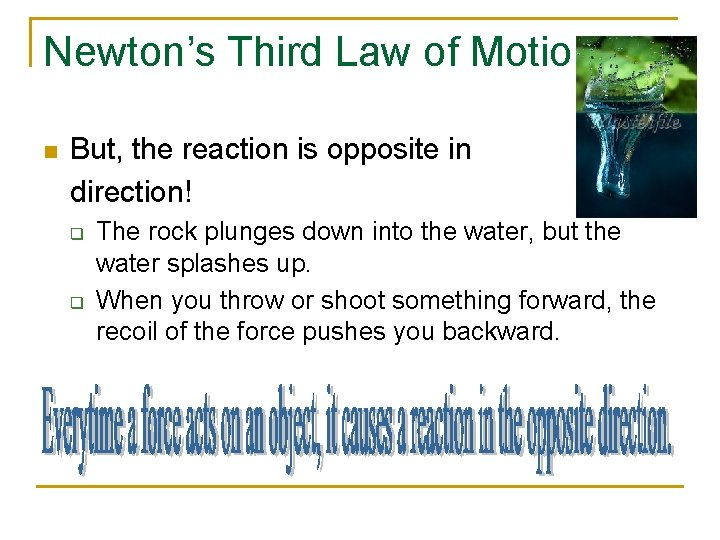Newton’s Third Law of Motion n But, the reaction is opposite in direction! q q The rock plunges down into the water, but the water splashes up. When you throw or shoot something forward, the recoil of the force pushes you backward.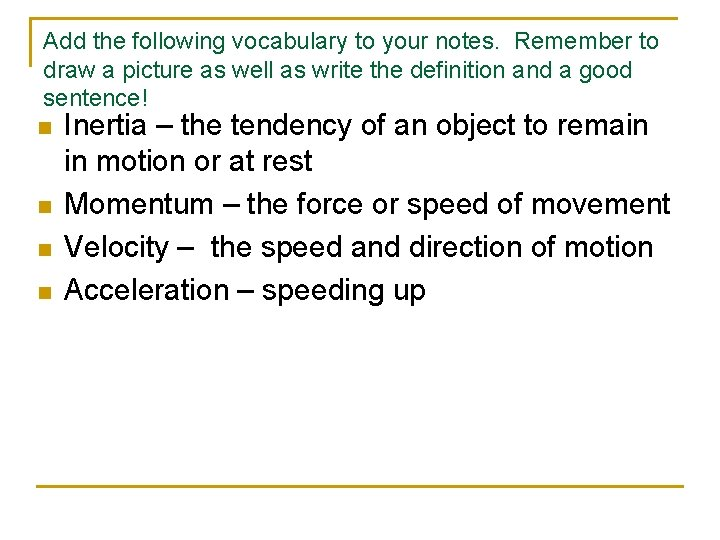Add the following vocabulary to your notes. Remember to draw a picture as well as write the definition and a good sentence! n n Inertia – the tendency of an object to remain in motion or at rest Momentum – the force or speed of movement Velocity – the speed and direction of motion Acceleration – speeding upNewton’s Laws of Motion n Law of Inertia – an object at rest tends to stay and an object in motion tends to stay in motion with the same speed and in the same direction unless some force interferes with its motion. Law of Acceleration – The greater the force, the greater the acceleration. Law of Action/Reaction – For every action, there is an equal and opposite reaction.